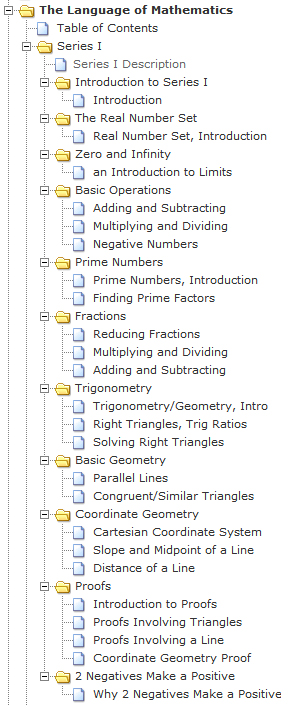| | | | |

## Monday, April 9, 2012

### Series I Description: What's Contained in this Series

The first part of this series deals with the absolute basics of mathematics: the Real Number Set; basic operations; prime numbers and their importance; and how to deal with fractions. This is where it all begins. Please be comfortable with this material before moving on.

The second part of this series deals with basic geometry and trigonometry. Topics discussed include: right triangles and trigonometric ratios; parallel lines; congruent and similar triangles; the Cartesian coordinate system; and slope, midpoint, and distance of a line. We also take a quick look at three different types of proofs related to these topics; those related to triangles, lines, and the Cartesian coordinate system. (NOTE: at some point in the future an in-depth introduction to trigonometry will be produce.)

In addition to the above, we take a rudimentary look at Zero and Infinity, and Why a Negative and a Negative Makes a Positive.

Full list and links for all the videos contained in the series are provided below and are available at: Table of Contents: The Language of Mathematics - Series I.

Exercises and Solutions are also available for this series.

See Videos Available for Download for information on the torrent for this series.

For ease of reference, included below is the Table of Contents and the expanded image of the tree menu provided in the left column of this site. Specific topics can be found through the Index (left column).

Section 1: Introduction to Series I
Section 2: The Real Number Set
Section 3: Zero and Infinity
Section 4: Basic Operations
Section 5: Prime Numbers
Section 6: Fractions
Section 7: Trigonometry
Section 8: Basic Geometry
Section 9: Coordinate Geometry
Section 10: Proofs
Section 11: Why a Negative and a Negative Makes a PositiveFrom Series I Tree Menu - The Language of Mathematics